# Difference between revisions of "Crossed module"

A morphism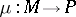of groups together with an action of the groupon the groupsatisfying two conditions which makes the structure a common generalization of:

1) a normal subgroup of;

2) a-module (cf. also Module);

3) the inner automorphism mapping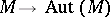;

4) an epimorphismwith central kernel. The two conditions are:for alland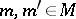, whereis the action ofon.

The notion is due to J.H.C. Whitehead [a9] and plays an important role in the theory of algebraic models of homotopy types of spaces.

In topology the structure arises from the second relative homotopy group and its boundary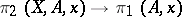[a5], or asfor a fibration. The second example has been used in relative algebraic-theory, to give a crossed module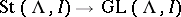for an idealin a ring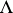.

From the first of these examples, crossed modules can be seen as-dimensional versions of groups, with,as, respectively, the- and-dimensional parts. This impression is confirmed by the existence of a functorial classifying space functor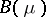of such a crossed module whose homotopy groups are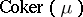,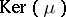in dimensionsandand are otherwise. For any connected pointed CW-complexthere are a crossed moduleand a mapping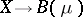inducing an isomorphism in homotopy in dimensionsand. This results from a homotopy classification of mappings. Thus, crossed modules capture all homotopy-types. These results are special cases of results on crossed complexes (cf. also Crossed complex).

It was proved in [a2] that the homotopy crossed module functor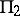from pointed pairs of spaces to crossed modules satisfies a generalized Van Kampen theorem, in that it preserves certain colimits. This allows for the determination of certain second relative homotopy groups as crossed modules, thus giving non-trivial non-Abelian information and often determining the-type of a space. Some of the explicit calculations are conveniently done by computer [a8].

One consequence of the generalized Van Kampen theorem is a result of J.H.C. Whitehead [a9], giving a certain second relative homotopy group as a free crossed module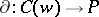: this is determined by a function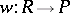from a setto the groupand satisfies a universal property. A common situation is whenis the free group on a set, andis an inclusion: then the free crossed module is determined by the presentation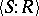of the group, andis known as the-module of identities among relations [a4], [a5] for the presentation. This module should be thought of as giving a non-Abelian form of syzygies (cf. also Syzygy), and as the start of a free crossed resolution of the group. Free crossed modules are also conveniently seen as special cases of crossed modules induced from a crossed module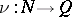by a morphism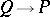of groups [a2], [a8].

There are a number of other algebraically defined categories equivalent to the category of crossed modules, namely: group objects in groupoids; groupoid objects internal to the category of groups; group objects internal to the category of groupoids; cat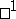-groups; simplicial groups whose Moore complex is of length(cf. also Simplicial complex); and certain kinds of double groupoids. This last fact is crucial in the proof of the generalized Van Kampen theorem in [a2], which uses a homotopy double groupoid of a pair of spaces. It is important to note that group objects internal to the category of groups are just Abelian groups: thus, the extension to groupoid objects in groups, or groupoid objects in groupoids, opens up the area of higher-dimensional non-Abelian structures for modeling geometry, and this has been exploited by a number of mathematicians. This seems to answer a dream of the topologists of the early part of the 20th century, of finding a higher-dimensional but still non-commutative analogue of the fundamental group.

Generalizing the above to the case whereis a groupoid rather than group yields the notion of crossed module of groupoids. This is important for many applications, and is the convenient format also for the generalized Van Kampen theorem. Such crossed modules are equivalent to-groupoids [a3].

The notion of groupoid object internal to the category of groups generalizes to groupoid object in other categories, and in many cases (basically when quotients are determined by kernels) one obtains a corresponding notion of crossed module [a7]. Such a notion is at the root of the area of non-Abelian homological algebra, see for example [a1], [a6].

A crossed module of groupoidshas a classifying space, and this gives a connection with homotopy-types (see [a10] for details and references).